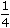# stocks and shares aptitude questions

11. A man invested Rs. 1552 in a stock at 97 to obtain an income of Rs. 128. The dividend from the stock is:
 A. 7.5% B. 8% C. 9.7% D. None of these

Explanation:

By investing Rs. 1552, income = Rs. 128.

 By investing Rs. 97, income = Rs.128 x 97= Rs. 8. 1552Dividend = 8%

12. A 12% stock yielding 10% is quoted at:
 A. Rs. 83.33 B. Rs. 110 C. Rs. 112 D. Rs. 120

Explanation:

To earn Rs. 10, money invested = Rs. 100.

 To earn Rs. 12, money invested = Rs.100 x 12= Rs. 120. 10Market value of Rs. 100 stock = Rs. 120.

13. The market value of a 10.5% stock, in which an income of Rs. 756 is derived by investing Rs. 9000, brokerage being%, is:
 A. Rs. 108.25 B. Rs. 112.20 C. Rs. 124.75 D. Rs. 125.25

Explanation:

For an income of Rs. 756, investment = Rs. 9000.

 For an income of Rs. 21 , investment = Rs.9000 x 21= Rs. 125. 2 756 2For a Rs. 100 stock, investment = Rs. 125.

 Market value of Rs. 100 stock = Rs.125 – 1= Rs. 124.75 4

14.

 The cost price of a Rs. 100 stock at 4 discount, when brokerage is 1 % is: 4
 A. Rs. 95.75 B. Rs. 96 C. Rs. 96.25 D. Rs. 104.25

Explanation:

 C.P. = Rs.100 – 4 + 1= Rs. 96.25 4

15. Sakshi invests a part of Rs. 12,000 in 12% stock at Rs. 120 and the remainder in 15% stock at Rs. 125. If his total dividend per annum is Rs. 1360, how much does he invest in 12% stock at Rs. 120?
 A. Rs. 4000 B. Rs. 4500 C. Rs. 5500 D. Rs. 6000

Explanation:

Let investment in 12% stock be Rs. x.

Then, investment in 15% stock = Rs. (12000 – x).12 x x + 15 x (12000 – x) = 1360. 120 125x + 3 (12000 – x) = 1360. 10 255x + 72000 – 6x = 1360 x 50x = 4000.